output.to from Sideway
Draft for Information Only

# Content

```Equivalent Couples Couples Translation  Forces Translation  Couples Rotation```

# Equivalent Couples

The moment of two couples are equal when the two force couples lie in two parallel planes or the same plane and they have equal moment of the same sense.imply the magnitude of the moment of the couples:## Couples Translation

Since the moment of a force couple is a free vector, two couples are equivalent when the force couple can be transformed by the translation of the force couple in space.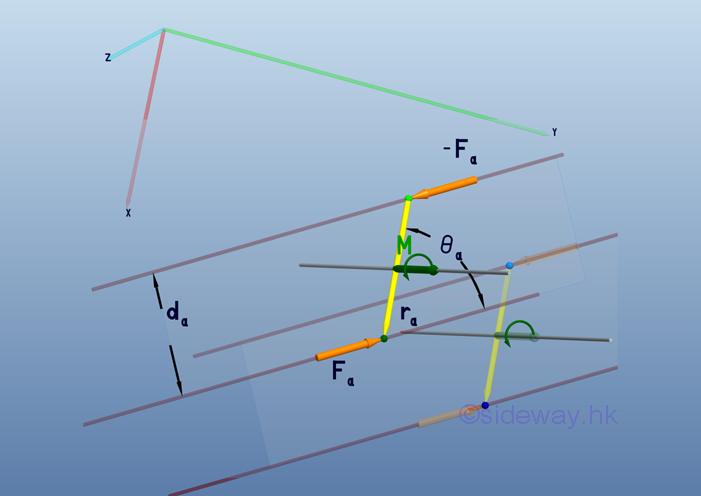Geometrically, both the forces of the couple and the moment arm are parallel to each other have the same gradient and magnitude after the couple translation. ImplyThe moment vector can be expressed as: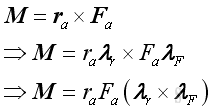Imply the moment vector depends on the magnitudes and the cross product of the direction vectors of the force couple only.

## Forces Translation

By the principle of transmissibility, two couples are equivalent when the force couples can be transfromed by the translation of the force components of a couple along its line of action without altering its magnitude and sense.Geometrically, the forces of the couple are parallel to each other have the same gradient and magnitude. Although the moment arm is changed as the forces of couple is translated along the lines of action, the prependicular distance between the forces of couple remains unchange. Imply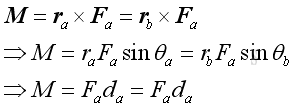Assume the moment arm vector ra after the translation of forces +m and -n along the line of action is rb. imply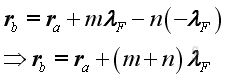The moment vector can be expressed as:## Couples Rotation

The moment of a force couple is a free vector to define the tendency of rotating action, two couples are equivalent when the force couple can be transformed by the rotation of the force couple about a centre axis normal to the plane on which the force couple lies.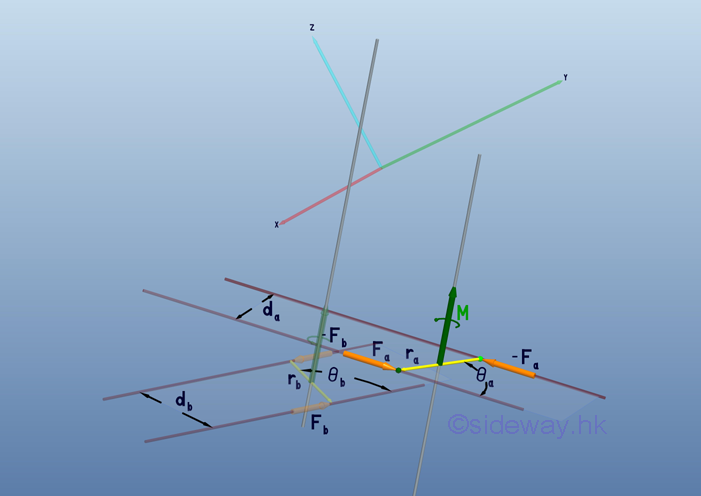Geometrically, the rotation of the force couple about the normal centre axis does not alter the relative relationship between the forces of couple and moment arm. As both the magnitude of forces of the couple and the prependicular distance between the line of action remain unchange, the moment is the same and independent of the angle of rotation. Imply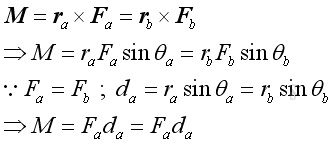To simplify the vector analysis, the force couple rotation can be reduced to 2D rotation by transformation through aligning the normal rotating centre axis  to the z axis of the rectangular coordinate.  imply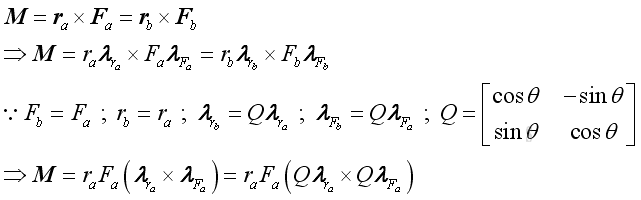The transformation of force couple rotation is: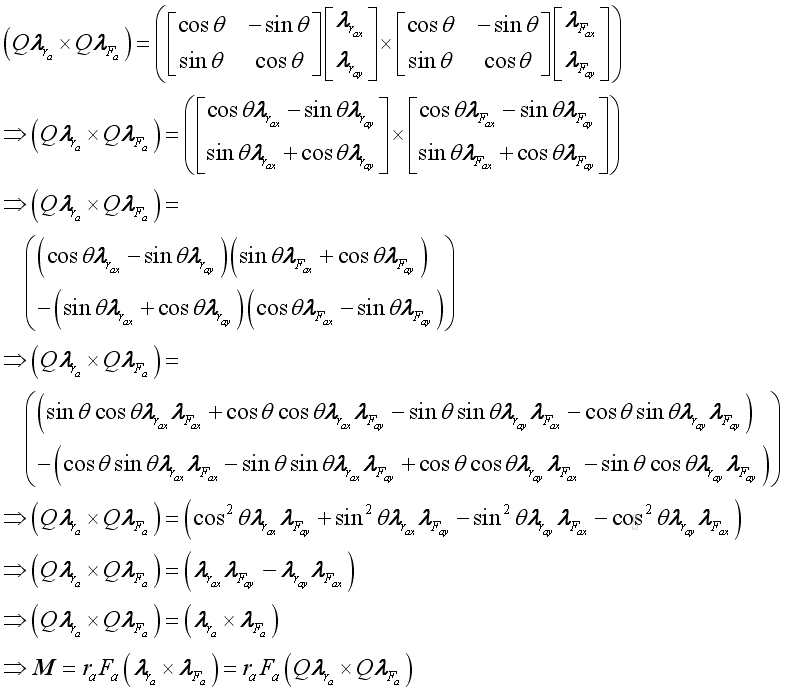Therefore the rotated force couple and the original force couple are equivalent.

Forces Rotation

By the parallelogram law, two couples are equivalent when the force couples can be transfromed by the decomposition of the force components of a couple into forces along its moment arm and the line of action of other force couple without altering its magnitude and sense. The rotated forces also lie on the plane of the force couple.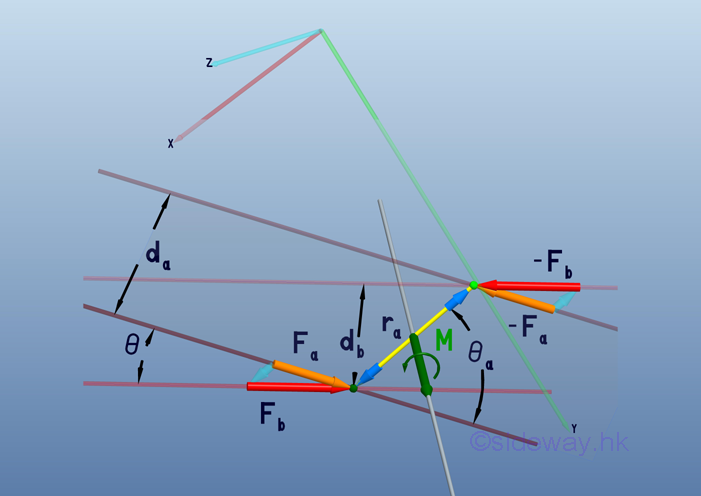The effective moment of both force couples can be expressed as:Geometrically, prependicular distances da and db can be expressed as: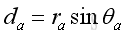andImply the prependicular distances db can be expressed in terms of da as:By the sine rule, force vectors Fb can be expressed in term of Fa  as: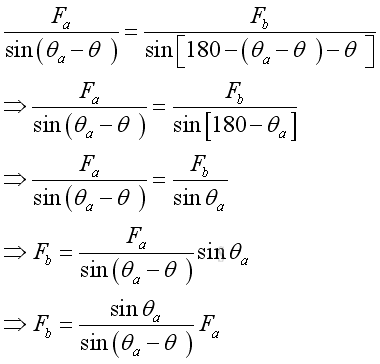Therefore the moment M is: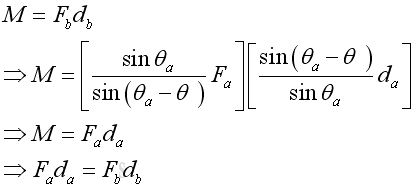The moment vector M can be expressed as: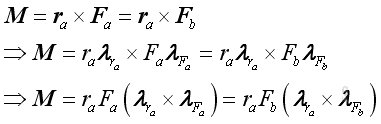To simplify the vector analysis, the rotation of the force of couple can be reduced to 2D rotation by transformation through aligning the rotating axis normal to plane of force couple at the point of application to the z axis of the rectangular coordinate.  implyTherefore the two couples are equivalent when the rotated force of the couple is at a specified ratio of the original force couple.

ID: 110600007 Last Updated: 6/28/2011 Revision: 0 Ref:References

1. I.C. Jong; B.G. rogers, 1991, Engineering Mechanics: Statics and Dynamics
2. F.P. Beer; E.R. Johnston,Jr.; E.R. Eisenberg, 2004, Vector Mechanics for Engineers: StaticsHome 5

Management

HBR 3

Information

Recreation

Culture

Chinese 1097

English 337

Computer

Hardware 154

Software

Application 207

Latex 35

Manim 203

Numeric 19

Programming

Web 285

Unicode 504

HTML 65

CSS 63

SVG 6

ASP.NET 210

OS 422

Python 64

Knowledge

Mathematics

Algebra 84

Geometry 25

Calculus 67

Engineering

Mechanical

Rigid Bodies

Statics 92

Dynamics 37

Control

Natural Sciences

Electric 27